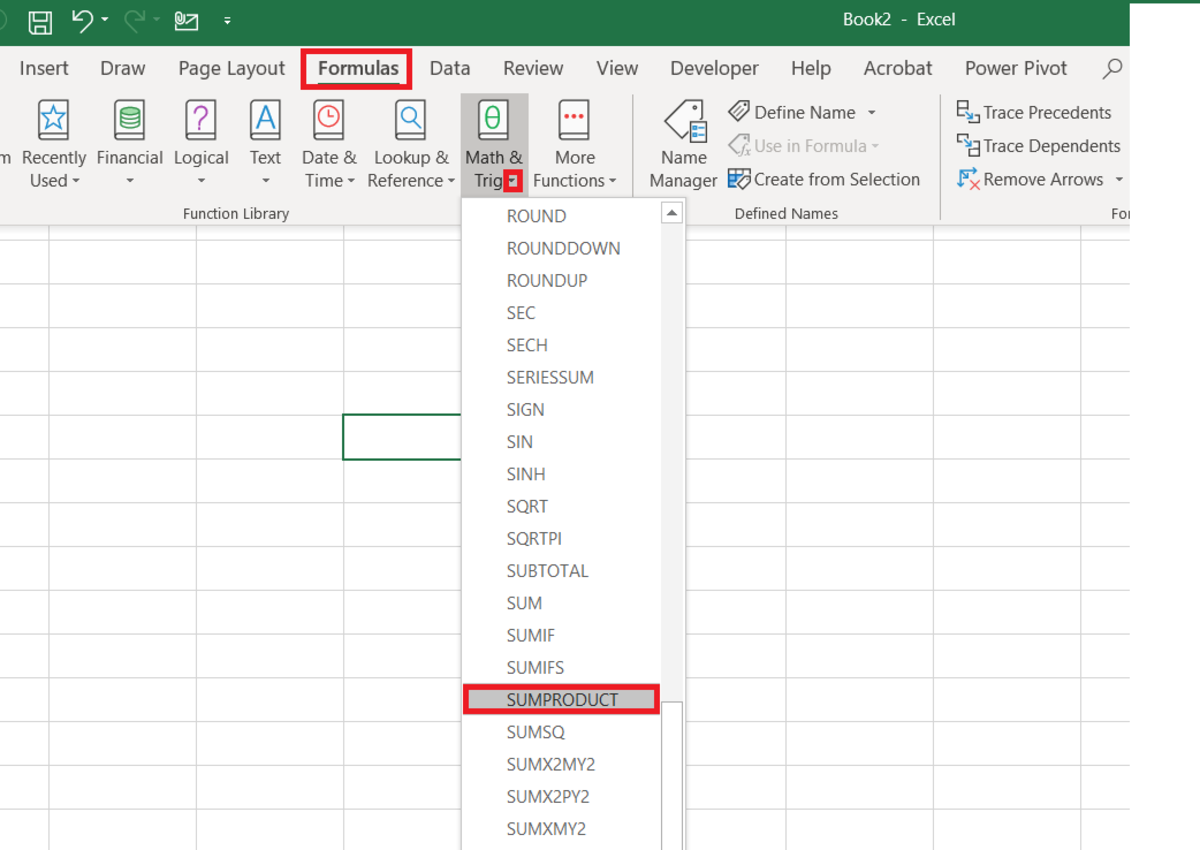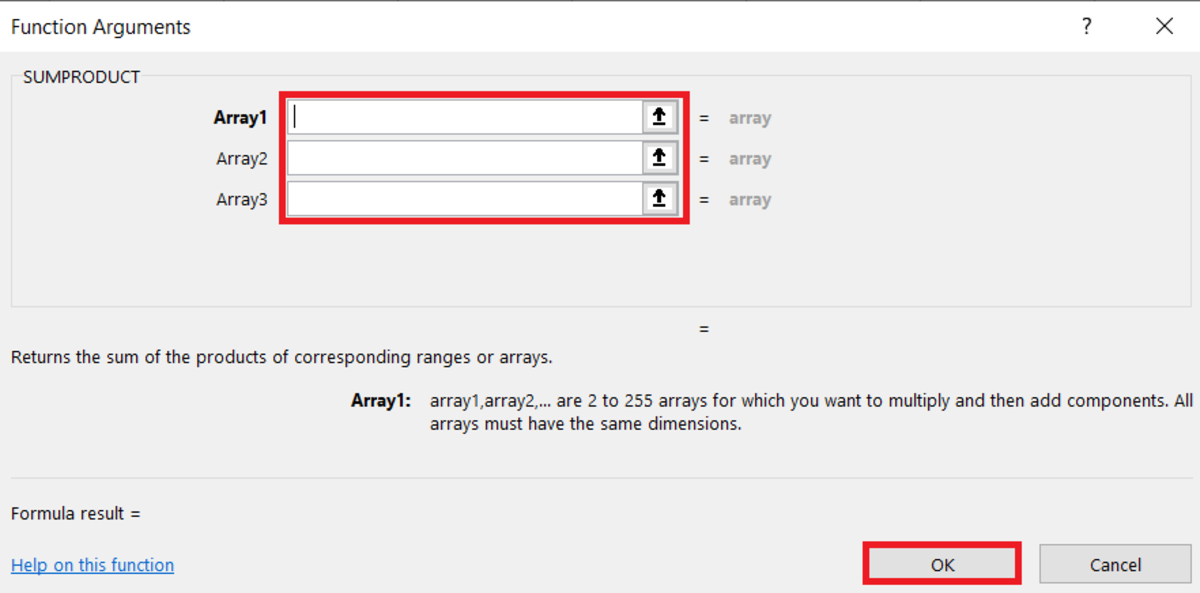# How to Use the SUMPRODUCT Function in ExcelThe SUMPRODUCT function multiplies arrays together while returning the sum of products.

Here the SUMPRODUCT function is discussed with a discussion of its purpose and its syntax. This will be followed by a simple example and a demonstration to show how it can be inserted into a spreadsheet.

## The Purpose of the SUMPRODUCT Function

The SUMPRODUCT function will return the sum of the products of ranges or arrays that correspond to each other. In other words, data from each cell in multiple ranges or arrays of the same size are multiplied together and then summed. The result appears in one cell.

If the two ranges used were A1:A2 and B1:B2, the result mathematically would come from the following equation:

[(A1)(B1)] + [(A2)(B2)]

## The SUMPRODUCT Function Syntax

The SUMPRODUCT function needs to be inputted into a cell like a formula to work. Manually add the SUMPRODUCT formula with the following steps:

1. First, a cell needs to be clicked in. Type "=SUMPRODUCT("

2. After the open parenthesis, the data (range, named range, cell references) that needs to be multiplied and summed needs to be entered with data inputs separated by commas.

3. This is then followed by a closing parenthesis. After the formula is created, the enter button can be pressed to display a result.

Below in bold is the syntax of the function with explanations of each argument.

=SUMPRODUCT(array1, array2)

The SUMPRODUCT function syntax has the following arguments:

array1 At least 1 array is required. This first array argument is the component that will be multiplied and then added.

array2 Any additional arrays added are optional. There can be 2 to 255 arrays in this function where each array will multiply and then add.

## SUMPRODUCT Example: Finding Total Order Cost

Here we illustrate a simple example of how the SUMPRODUCT function can be used. Suppose that you have an order of multiple items in a spreadsheet. If you have the unit price, and the quantity for the order you should be able to calculate the total order cost with this function.

Take for instance the example below.

The SUMPRODUCT function can be used to find the total cost of each item above and display the sum of all of those individual costs. The function is shown below after selecting each array of data.

Here the result is displayed in the cell.

## Inserting the SUMPRODUCT Function

The SUMPRODUCT function can be inserted into a cell with the use of an insert tool from the formulas tab with the following steps:

1. To start this process, select and click on a cell.
2. Click on the formulas tab and click on the "Math & Trig" button.
3. The SUMPRODUCT selection needs to be chosen from the drop-down menu.To insert the SUMPRODUCT function from the formulas tab you must first navigate to Formulas→Math & Trig→SUMPRODUCT.

After the function arguments window appears, all arrays that need to be multiplied and summed can be added to the fields. The results can be been after arguments are added. After the arrays are added, the OK button can be selected.

## The Function Arguments WindowIn this window, the function arguments can be added to the function. After an argument field is clicked, instructions will appear and data can be entered.

This content is accurate and true to the best of the author’s knowledge and is not meant to substitute for formal and individualized advice from a qualified professional.